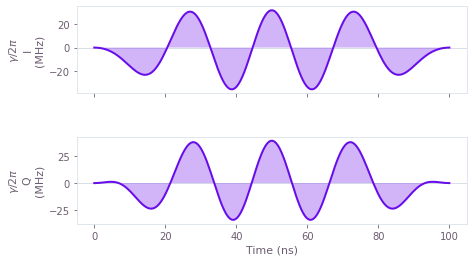# How to perform model-based optimization with user-defined basis functions

Create optimized controls using arbitrary basis functions

The Q-CTRL Python package exposes a highly-flexible optimization engine for general-purpose gradient-based optimization. The pulses can be described in terms of optimizable linear combinations from a set of user-defined basis functions, which can greatly reduce the dimensionality of the optimization search space. In this notebook we will use Hanning window functions, although the same technique has also seen success with other bases, for example Slepian functions. For an optimization using Fourier basis, check this user guide.

## Summary workflow

### 1. Define basis function for signal composition in the graph

The Q-CTRL optimization engine provides a library of convenience functions, qctrl.operations, for creating optimizable signals in a custom basis. In the worked example below, the decomposition of the pulse in terms of the chosen basis is defined by the function custom_function.

### 2. Execute graph-based optimization

With the graph object created, an optimization can be run using the qctrl.functions.calculate_optimization function. The cost, the outputs, and the graph must be provided. The function returns the results of the optimization.

## Worked example: Robust optimization on a qutrit using Hanning window functions

In this example, we perform optimization for a robust single qubit Hadamard gate (in the Hanning window basis) of a qutrit system while minimizing leakage out of the computational subspace. The system is described by the following Hamiltonian:

\begin{align*} H(t) = & \frac{\chi}{2} (a^\dagger)^2 a^2 + (1+\beta(t)) \left(\gamma(t) a + \gamma^*(t) a^\dagger \right), \end{align*}

where $\chi$ is the anharmonicity, $\gamma(t)$ is a complex time-dependent pulse, $\beta(t)$ is a small, slowly-varying stochastic amplitude noise process, and $a = |0 \rangle \langle 1 | + \sqrt{2} |1 \rangle \langle 2 |$.

In this example, we will parametrize the pulse $\gamma(t) = \gamma_I(t) + i \gamma_Q(t)$ in terms of Hanning window functions:

\begin{align*} \gamma_{I(Q)}(t) = \sum_{n=1}^N{\frac{c^{I(Q)}_n}{2} \left[1-\cos\left(\frac{2\pi nt}{\tau_g}\right) \right]}, \end{align*}

where $c^{I(Q)}_n$ are the different real-valued coefficients describing the parametrization and $\tau_g$ is the gate duration. This is a good choice for implementation in bandwidth-limited hardware as it is composed of smooth functions that go to zero at the edges.

import matplotlib.pyplot as plt
import numpy as np

from qctrlvisualizer import plot_controls
from qctrl import Qctrl

# Starting a session with the API
qctrl = Qctrl()


First, define the operators and parameters for the optimization:

# Define standard matrices
a = np.diag([1.0, np.sqrt(2.0)], k=1)
[[1.0, 1.0, 0], [1.0, -1.0, 0], [0, 0, np.sqrt(2)]], dtype=complex
) / np.sqrt(2)
qubit_projector = np.pad(np.eye(2), ((0, 1), (0, 1)), mode="constant")

# Define physical constraints
chi = 2 * np.pi * -300.0 * 1e6  # Hz
gamma_max = 2 * np.pi * 50e6  # Hz
segment_count = 200
duration = 100e-9  # s
sample_times = np.linspace(0, duration, segment_count)


Next, define the function to generate the pulse components $\gamma_I(t)$ and $\gamma_Q(t)$ in terms of Hanning windows. The inputs are: the time as a sampleable time-dependent function (STF), the gate duration $\tau_g$, and the total number $N$ of Hanning window functions in the decomposition.

def custom_function(duration, optimizable_frequency_count):

time = qctrl.operations.identity_stf()

# define the coefficients of the Hanning functions for optimization
hanning_coefficients = qctrl.operations.optimization_variable(
optimizable_frequency_count, lower_bound=-1, upper_bound=1
)
frequency = 2.0 * np.pi / duration

gamma = qctrl.operations.stf_sum(
[
0.5
* hanning_coefficients[index - 1]
* (1 - qctrl.operations.cos(time * index * frequency))
for index in np.arange(1, optimizable_frequency_count + 1)
]
)
return gamma


Finally, create and execute the optimization graph:

optimizable_frequency_count = 5

# Create graph object
with qctrl.create_graph() as graph:

# Create gamma(t) signal in Hanning function basis
gamma_i = custom_function(duration, optimizable_frequency_count)
gamma_q = custom_function(duration, optimizable_frequency_count)

gamma = gamma_max * (gamma_i + 1j * gamma_q)

# Discretize gamma to export and plot
sample_gamma = qctrl.operations.discretize_stf(
stf=gamma, duration=duration, segment_count=segment_count, name=r"$\gamma$"
)

# Create anharmonicity term
anharmonicity = ad2a2 * chi / 2

# Create drive term
drive = qctrl.operations.stf_operator_hermitian_part(2 * gamma * a)

# Create target operator in qubit subspace
target_operator = qctrl.operations.target(
)

# Create infidelity
infidelity = qctrl.operations.infidelity_stf(
hamiltonian=anharmonicity + drive,
target=target_operator,
sample_times=sample_times,
noise_operators=[drive],
name="infidelity",
)

# Run the optimization and retrieve results
optimization_result = qctrl.functions.calculate_optimization(
cost_node_name="infidelity", output_node_names=[r"$\gamma$"], graph=graph
)

print(f"\nOptimized cost:\t{optimization_result.cost:.3e}")

plot_controls(plt.figure(), optimization_result.output, smooth=True, polar=False)

Your task calculate_optimization (action_id="533708") has started. You can use the qctrl.get_result method to retrieve previous results.